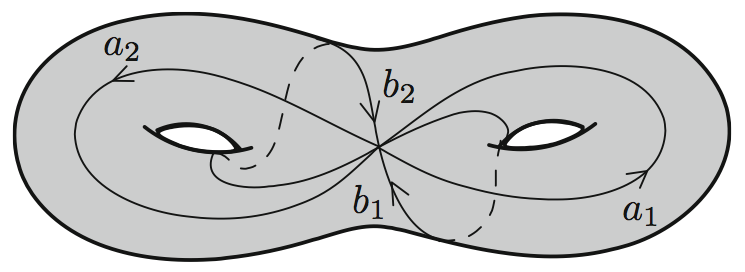Chris Swierczewski's Website

# Clawpack Lightning Talk Introduction

Jul 22, 2013

I'm a student in the Applied Mathematics depatment at the University of Washington. My research is in "computational complex algebraic geometry". I do a mixture of symbolic and numerical computation.

• Special functions.
• Algebraic curves. (Bi-variate polynomial.)
• Algebra and geometry.

$f(x,y) = y^3 + 2x^3y - x^7$Cartoon of complex topology of f(x,y)=0f(x,y) = 0f(x,y)=0. (You need 4-dimensional eyes to actually visualize it.)

Abelian functions: higher dimensional versions of complex periodic functions.

• Defined on algebraic curves.
• All Abelian functions are built from Riemann theta functions.
• Periodic solutions to integrable partial differential equations are dense in space of Abelian functions.
• Applications in optimization and algebraic geometry as well.

I have some background in high performance computing.

• Special functions: Riemann theta function.
• Computational number theory.
• GPU / CUDA.

I'm working on a project with Daniel Shapero on a FORTRAN-to-C wrapper called f2c. (Similar idea to Cython for C-to-Python.) However, I'm happy to help with anything!